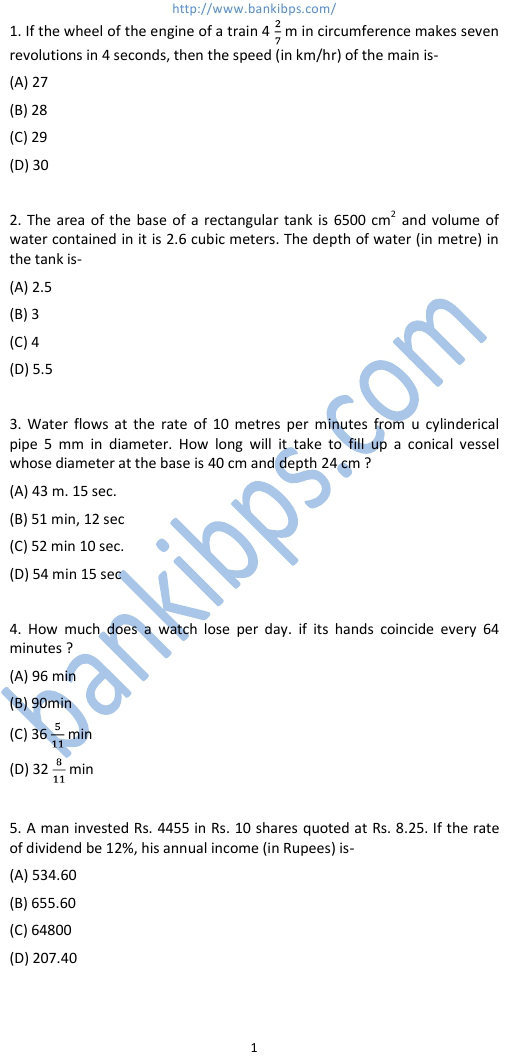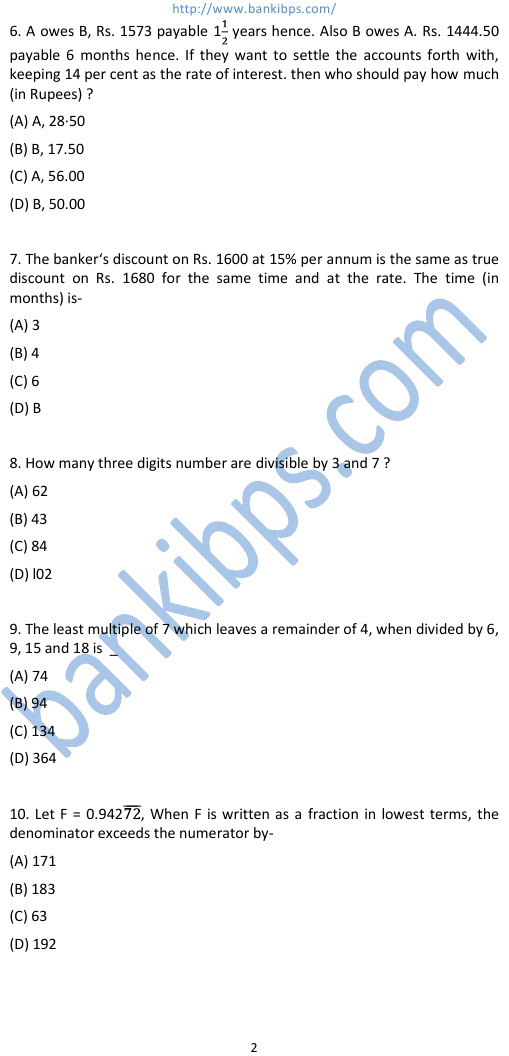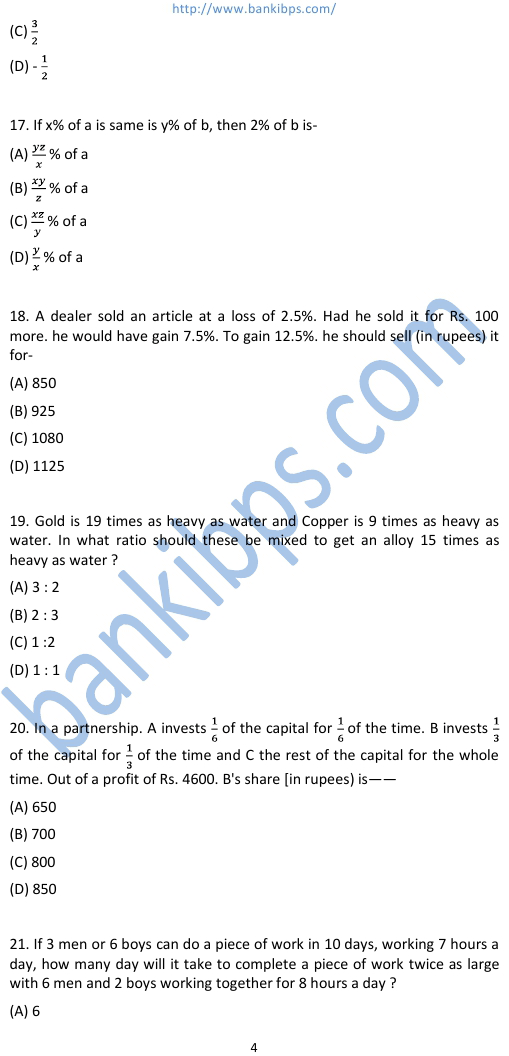# LIC of India Agent Exam Question Paper

1. If the wheel of the engine of a train 4 2/7 m in circumference makes seven revolutions in 4 seconds, then the speed (in km/hr) of the main is- (A) 27 (B) 28 (C) 29 (D) 30 2. The area of the base of a lic of india agent exam question paper rectangular tank is 6500 cm2 and volume of water contained in it is 2.6 cubic meters. The depth of water (in metre) in the tank is- (A) 2.5 (B) 3 (C) 4 (D) 5.5 3. Water flows at the rate of 10 metres per minutes from u cylinderical pipe 5 mm in diameter. How long will it take to fill up a conical vessel whose diameter at the base is 40 cm and depth 24 cm ? (A) 43 m. 15 sec. (B) 51 min, 12 sec (C) 52 min 10 sec. (D) 54 min 15 sec 4. How much does a watch lose per day. if its hands coincide every 64 minutes ? (A) 96 min (B) 90min (C) 36 5/11 min (D) 32 8/11 min 5. A man invested Rs. 4455 in Rs. 10 shares quoted at Rs. 8.25. If the rate of dividend be 12%, his annual income (in Rupees) is- (A) 534.60 (B) 655.60 (C) 64800 (D) 207.40## lic of india agent exam question paper

### lic test question papers

#### lic agent test papers

##### lic test paper
###### lic agents exam model question paper
lic test question papers. lic agent test papers. lic test papers agents. lic test paper. lic agent model question paper. lic agent exam model question paper. lic agents exam model question paper. lic exam model question paper. lic model question papers. lic model question paper. sample paper for lic agent exam. lic agent exam sample paper. lic sample question papers. lic exam sample question paper.